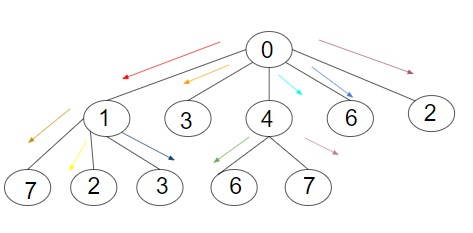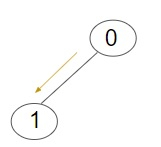# Minimum no. of iterations to pass information to all nodes in the tree in C++

C++Server Side ProgrammingProgramming

We are given a tree data structure with ‘n’ number of nodes. The given tree will have a root node and respective children which can be any number and further child can have any number of children. The task is to find the minimum number of iterations required by a root node of a tree to pass the information to all nodes in a tree. At a time, a node can pass information to one of its children and further one of its children can pass information to one of its children and meanwhile root node can pass information to another child.

Let us see various input output scenarios for this -

In −Out −Minimum no. of iterations to pass information to all nodes in the tree are: 5

Explanation −We are given a tree with a total of 11 nodes including root and all other nodes. The root node of a given tree is 0 which will firstly pass the data to the node 1 as it has many children then other nodes, then root node will pass data to node 4, then it will pass data to 3, then it will pass data to 6 and at last it will pass data to 2. So, in total the iterations required are 5.

In −Out − Minimum no. of iterations to pass information to all nodes in the tree are: 1

Explanation −: We are given a tree with a total of 2 nodes including root and all other nodes. Since there is only one child of a root node in a given tree so the minimum number of iterations required will be 1.

## Approach used in the below program is as follows −

• Create a class to construct the tree and add nodes as its data member and create a list pointer as List_children and declare a private method as void Iteration(int vertices, int arr[]). Declare a parameterised constructor as Tree(int nodes), void insert_node(int a, int b), int Min_Iteration() and static int check(const void *a_1, const void *b_1).

• Call an external parameterized constructor as Tree::Tree(int nodes)

• Set this->nodes to nodes.

• Set List_children to new list[nodes]

• Call a class method as void Tree::insert_node(int a, int b)

• Set List_children[a] to push_back(b)

• Call a class method as void Tree::Iteration(int vertices, int arr[])

• Set arr[vertices] to List_children[vertices].size()

• Set *ptr to new int[arr[vertices]]

• Set temp to 0 and temp_2 to 0

• Declare an iterator as list::iterator it

• Start loop FOR from it to List_children[vertices].begin() till it does not equals to List_children[vertices].end() and pre increment it. Inside the loop set Iteration(*it, arr) and set ptr[temp++] to arr[*it]

• Call qsort(ptr, arr[vertices], sizeof(int), check) for quick sort

• Start Loop FOR temp to 0 and temp less than List_children[vertices].size() and post increment temp. Inside the loop, set temp_2 to ptr[temp] + temp + 1 and arr[vertices] to max(arr[vertices], temp_2) and delete[] ptr

• Call a class method as int Tree::Min_Iteration()

• Declare a pointer as int *ptr = new int[nodes]

• Declare a variable as int temp = -1

• Start loop FOR from i to 0 till i < nodes and i++. Inside the loop, set ptr[i] to 0

• Call Iteration(0, ptr) and set temp to ptr and delete[] ptr

• return temp

• Call a class method as int Tree::check(const void * a_1, const void * b_1)

• Declare a variable as int result to ( *(int*)b_1 - *(int*)a_1 )

• return result

• In the main() function

• Create a tree object using a parameterized constructor.

• Then using the object of a tree class call the insert_node() method to insert the node data to the tree

• Call Min_Iteration() method to calculate the minimum number of iterations to pass information to all nodes in the tree

## Example

#include<bits/stdc++.h>
using namespace std;

class Tree
{
int nodes;
list<int> *List_children;
void Iteration(int vertices, int arr[]);

public:
//constructor of a class
Tree(int nodes);
//method to insert a node in a tree
void insert_node(int a, int b);
//method to calculate the minimum iterations
int Min_Iteration();
static int check(const void *a_1, const void *b_1);
};

Tree::Tree(int nodes)
{
this->nodes = nodes;
List_children = new list<int>[nodes];
}
void Tree::insert_node(int a, int b)
{
List_children[a].push_back(b);
}
void Tree::Iteration(int vertices, int arr[])
{
arr[vertices] = List_children[vertices].size();
int *ptr = new int[arr[vertices]];
int temp = 0;
int temp_2 = 0;
list<int>::iterator it;

for(it = List_children[vertices].begin(); it!= List_children[vertices].end(); ++it)
{
Iteration(*it, arr);
ptr[temp++] = arr[*it];
}

qsort(ptr, arr[vertices], sizeof(int), check);

for(temp = 0; temp < List_children[vertices].size(); temp++)
{
temp_2 = ptr[temp] + temp + 1;
arr[vertices] = max(arr[vertices], temp_2);
}
delete[] ptr;
}
int Tree::Min_Iteration()
{
int *ptr = new int[nodes];
int temp = -1;

for (int i = 0; i < nodes; i++)
{
ptr[i] = 0;
}
Iteration(0, ptr);
temp = ptr;
delete[] ptr;
return temp;
}
int Tree::check(const void * a_1, const void * b_1)
{
}
int main()
{
Tree T_1(8);
T_1.insert_node(0, 1);
T_1.insert_node(0, 3);
T_1.insert_node(0, 4);
T_1.insert_node(0, 6);
T_1.insert_node(0, 2);
T_1.insert_node(1, 7);
T_1.insert_node(1, 2);
T_1.insert_node(1, 3);
T_1.insert_node(4, 6);
T_1.insert_node(4, 7);
cout<<"Minimum no. of iterations to pass information to all nodes in the tree are:"<<T_1.Min_Iteration();

Tree T_2(2);
T_2.insert_node(0, 1);
cout<<"\nMinimum no. of iterations to pass information to all nodes in the tree are:" <<T_1.Min_Iteration();

return 0;
}

## Output

If we run the above code it will generate the following Output

Minimum no. of iterations to pass information to all nodes in the tree are: 8
Minimum no. of iterations to pass information to all nodes in the tree are: 8